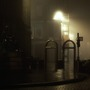M e s s a g e s t o t h e S l e e p w a l k e r ( P a r t 1 )250
•
4

Autoplay OFF   •   a year ago
Part 1 is out now! Low-key don't know what it is or how it will go on, but it's kind of real life inspired and I'll be just as in the dark as you. Stay tuned!

# M e s s a g e s t o t h e S l e e p w a l k e r ( P a r t 1 )

✧･ﾟ: *✧･ﾟ:* ✧･ﾟ: *✧･ﾟ:* ✧･ﾟ: * Chapter 1 'Liar'

F a m n e r g e t d

F a m n e r g e t d b e t w e e n

F a m n e r g e t d b e t w e e n different

F a m n e r g e t d b e t w e e n i

F a m n e r g e t d b e t w e e n tiniest

F a m n e r g e t d b e t w e e n tiniest of places

F a m n e r g e t d b e t w e e no tiniest of places

F a m n e r g e t d b e t w e e no common tiniest ground of places

F a m n e r g e t d b e t w e e no common tiniest ground of almost places

F a m n e r g e t d b e t w e e nothing common tiniest ground of almost places

F a m n e r g e t d b e t w e e nothing common tiniest ground of almost places to hold onto

F a m n e r g e t d b e t w e e nothing common tiniest ground of almost places to hold onto I M B A L A N C E

I M B A L A N C E

in steps, I M B A L A N C E

transition in steps, of the soil to w a t e r I M B A L A N C E

in matters of less transition in steps, of the soil to w a t e r I M B A L A N C E

in matters of less transition than in steps, of the soil to w a t e r I M B A L A N C E

in matters of less SECONDS transition than in steps, of the soil to w a t e r I M B A L A N C E

is the instability in matters of less SECONDS transition than in steps, of the soil to w a t e r I M B A L A N C E

feel is the instability in matters of less SECONDS transition than in steps, of the soil to w a t e r I M B A L A N C E

feel is the instability in matters of less SECONDS transition than in steps, of the soil to w a t e r I M B A L A N C E

feel is the instability in matters of less SECONDS transition than in steps, of the soil to w a t e r I M B A L A N C E

feel is the instability in matters of less SECONDS transition than in steps, of the soil to w a t e r I M B A L A N C E

feel is the instability in matters of less SECONDS transition than in steps, of the soil to w a t e r I M B A L A N C E

fe is the instability e in matters of less l SECONDS transition than in steps, of the soil to w a t e r I M B A L A N C E

is the instability f in matters of less e SECONDS e transition than l in steps, of the soil to w a t e r I M B A L A N C E

is the instability in matters of less SECONDS f transition than in steps, e of the soil to w a t e r e I M B A L A N C E l

is the instability in matters of less SECONDS transition than in steps, of the soil to w a t e r I M B A L A N C E

followingclosebehind

followingclosebehind,

followingclosebehind, a s h a d o w

'Heyyyy, are you ok?'

'Heyyyy, are you ok?' Yea, yea, sorry, I just got a bit distracted

'Heyyyy, are you ok?' Yea, yea, sorry, I just got a bit distracted 'Hahahaha, if you say'

'Heyyyy, are you ok?' Yea, yea, sorry, I just got a bit distracted 'Hahahaha, if you say' Anyway, listen, I was thinking of calling it a night

'Ok' 'Hey, um'

'Ok' 'Hey, um' Yea? What is it?

'Ok' 'Hey, um' Yea? What is it? 'Nothing, nevermind'

'Ok' 'Hey, um' Yea? What is it? 'Nothing, nevermind' 'Goodnight, rest well'

Geez, obviously the worst liar ever.

Anyway, can't deal with it now. *sigh*

*sigh*

Stories We Think You'll Love 💕